Next: Count rate predictions for Up: Flux conversions Previous: Flux conversions

## General considerations

It is important to note that it can be extremely difficult to make accurate count rate predictions for the WFC, principally because it operates in the XUV, a waveband largely unexplored to date and in which absorption is very important. The following general considerations should be borne in mind before attempting any feasibility calculations:

1. Observations in the XUV are extremely sensitive to absorption in the inter-stellar medium (ISM). This is illustrated in Figure 12.2which shows the mean free path (i.e., distance for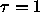) as a function of wavelength for an assumed mean ISM hydrogen number density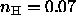cm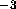(e.g., Paresce, 1984, AJ, 89, 1022), corresponding to a column density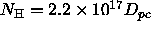cm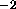, where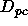is the source distance in parsec. At 125 eV (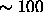Å) the mean free path is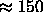pc, but it falls below 10 pc for E < 40 eV (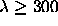Å). A detailed discussion of the ISM density distribution is inappropriate here, but it should be noted that it shows considerable spatial variation. Although there may be directions for which the mean column density is lower than assumed for Figure 12.2, there are certainly directions with substantially higher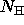.
2. The predicted count rate for the WFC is relatively sensitive to the form of the spectrum assumed. This presents problems if the appropriate spectral form is not known, since in the XUV band the differences between simplified spectral models (e.g., approximate thermal bremsstrahlung or black-body distributions described by only 2 parameters) and the `correct' description (e.g., detailed optically thin spectra or model atmospheres) can be much larger than at higher photon energies. Any predictions based on simple spectral models should therefore be treated with some caution.
3. Given these complications, it should be clear that simple extrapolations of results obtained at higher energies (e.g., with Einstein or EXOSAT) to the WFC band can be very misleading. Section 12.5gives some guidance on this point.

The conversion factors given in this section apply only to on-axis sources. For off-axis sources the WFC vignetting (Figure 5.3) will reduce the predicted count rate (although this is probably negligible for prediction purposes), and the degradation of the point source response (see § 12.8and Figures 5.4and 5.5) will increase the minimum detectable count rate (§ 12.2) since a larger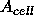size is implied (note however that the point source response is approximately constant within a radius of 1 degree from the centre of the field of view).Next: Count rate predictions for Up: Flux conversions Previous: Flux conversions

Michael Arida
Tue Jun 11 16:18:41 EDT 1996